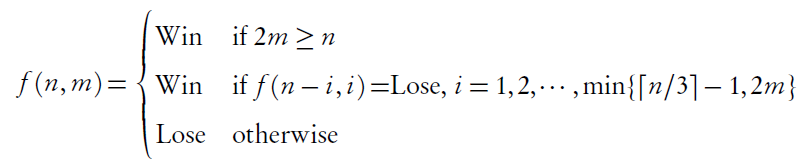# Fibonacci Nim取子游戏

## 问题描述

1. 第一次取石子时不能将所有石子取光。
2. 每一次至少取一个石子。
3. 每一次可以取的石子数不超过上一个人取的石子数的二倍。

## 寻找思路

• n=2 时： A取 1 个石子，到达状态 1；B取走 1 个石子。B获胜。即，先手必败。
• n=3 时： 若一开始，A取 1 个石子，到达状态 2；B取走 2 个石子；B获胜。若一开始A取 2 个石子，则B取走 1 个石子；B获胜。即，先手必败。
• n=4 时： 若一开始，A取 1 个石子，则B至多只能取 2 个石子，等同于当 n=3 时的A。因此，当 n=4 时，先手必胜。
• n=5 时： 一开始，若A取 1 个石子，则B相当于 n=4 时的A，B必胜；一开始，若A取 2 个石子，则B可以将剩下的 3 个石子取光，B必胜。因此，当 n=5 时，先手必败。
• n=6 时： 一开始，若A取 1 个石子，则B相当于 n=5 时的A，B必败；因此，当 n=6 时，先手必胜。

1. 原问题的子问题不仅与石子个数有关，还与上一个人取的石子个数有关。
2. 如果先手必败态具有显著的规律性的话，一定是因为有若干个“必败点”，一旦到达这些“必败点”，无论上一个人取了多少石子，轮到我们的时候都是必败的。

## 递归解int f(int n, int m)
{
if(2 * m >= n) {
return 1;
}
/* "(n + 2) / 3" in integer operation equals to
* "ceiling(n / 3) - 1" in float operation.
*/
int i = 1, bound = min((n + 2) / 3, 2 * m);
for(; i <= bound; i++) {
if(f(n - i, i) == 0) {
return 1;
}
}
return 0;
}

P  P  P  N  P  N  N  P  N  N
1  2  3  4  5  6  7  8  9 10
N  N  P  N  N  N  N  N  N  N
11 12 13 14 15 16 17 18 19 20
P  N  N  N  N  N  N  N  N  N
21 22 23 24 25 26 27 28 29 30
N  N  N  P  N  N  N  N  N  N
31 32 33 34 35 36 37 38 39 40
N  N  N  N  N  N  N  N  N  N
41 42 43 44 45 46 47 48 49 50

## 动态规划解

int f(int n, int m)
{
if(2 * m >= n) {
return 1;
}
if(cache[n][m] != NAN) {
return cache[n][m];
}
/* "(n + 2) / 3" in integer operation equals to
* "ceiling(n / 3) - 1" in float operation.
*/
int i = 1, bound = min((n + 2) / 3, 2 * m);
for(; i <= bound; i++) {
if(f(n - i, i) == 0) {
return cache[n][m] = 1;
}
}
return cache[n][m] = 0;
}

1   2   3   5   8  13  21  34  55  89
144 233 377 610 987

## 参考资料

1. 机械工业出版社《算法导论（原书第 2 版）》，第 15 章 动态规划，15.3 动态规划基础 做备忘录 P207。
2. EP8: Fibonacci Nim（斐波那契取石子博弈）
posted @ 2012-05-01 15:44  HCOONa  阅读(1413)  评论(0编辑  收藏  举报